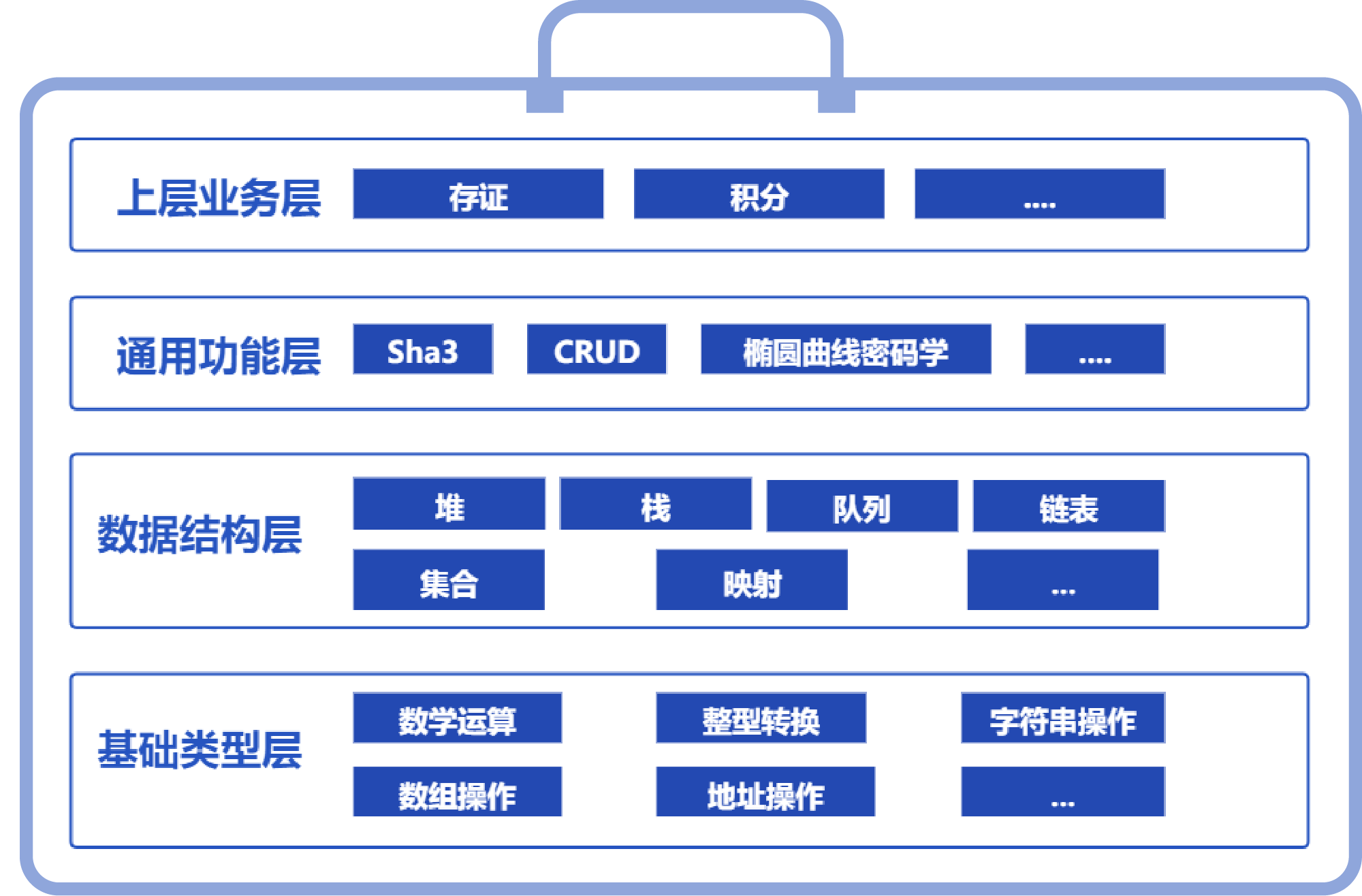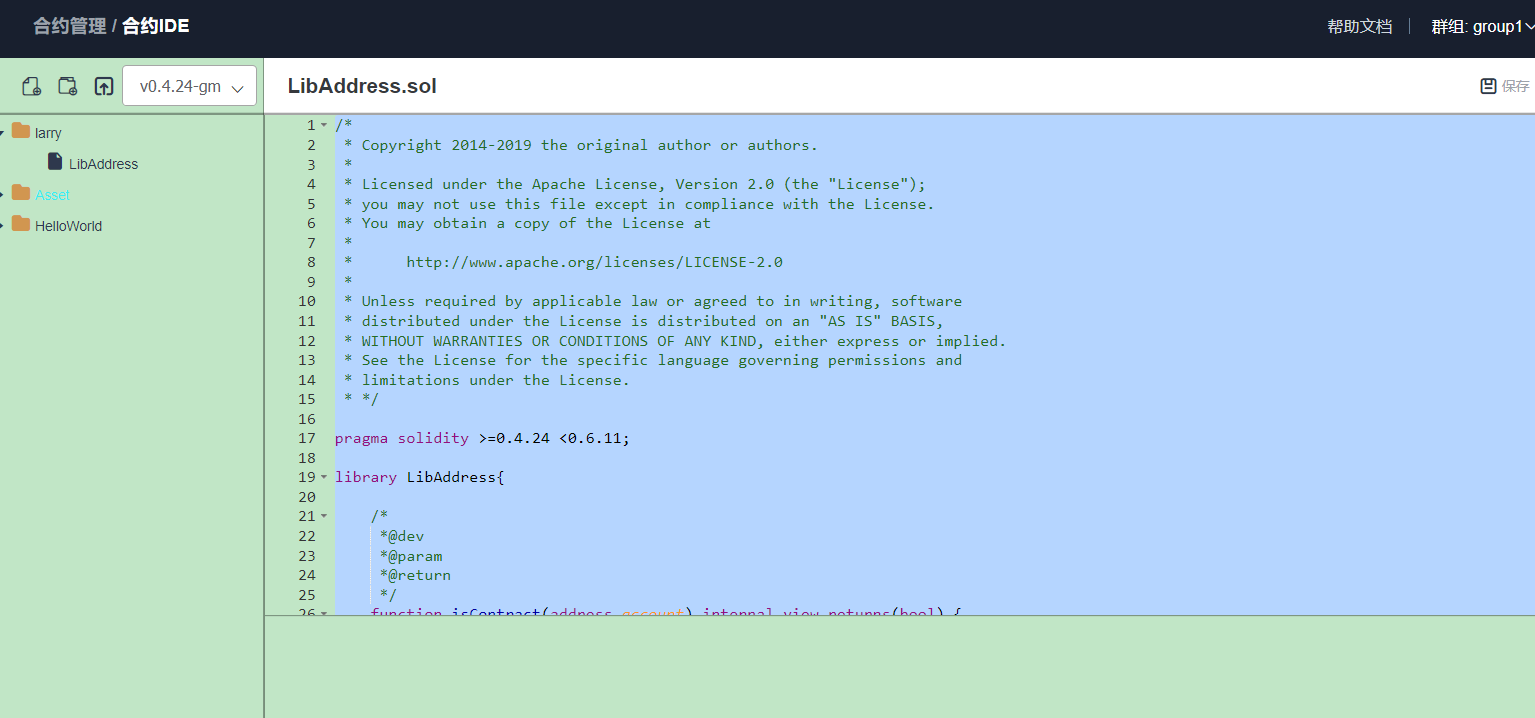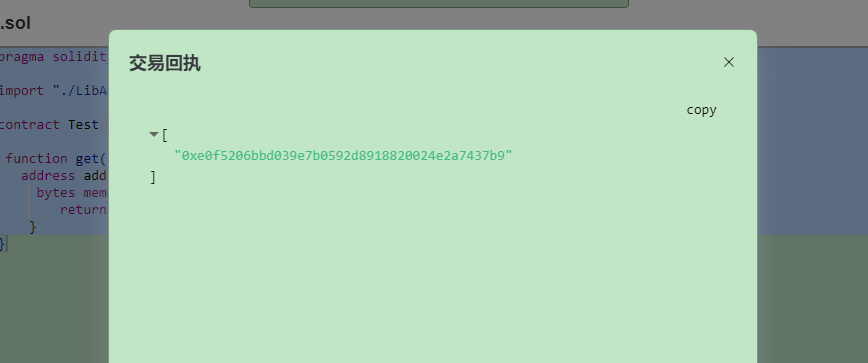# 微众银行《Solidity智能合约库》区块链工程师的随身工具箱之初体验

https://www.interchains.cc/21750.html

### 微众银行《Solidity智能合约库》区块链blockchain工程师的随身工具箱之初体验

• Solidity智能合约库
• Solidity工具

# 一、智能合约库简介

SmartDev-Contract智能合约库是一个“麻雀虽小，五脏俱全”的智能合约的工具类库，通过Solidity的library封装，旨在帮助Solidity开发者提升开发体验，避免重复造轮子，让编写Solidity语言也可以如编写Python语言那样“丝滑顺畅”。

SmartDev-Contract智能合约库的每个合约文件都来自于微众银行区块链blockchain工程师的细致打磨，来自于实际使用场景的“聚沙成塔”，覆盖了业务场景开发中的各种“犄角旮旯”，是开发智能合约的“10倍工程师”的不二法门。区块链blockchain工程师的随身工具箱之初体验” />

# 二、痛点及解决方式

``pragma solidity ^0.4.25; import "./LibAddress.sol"  contract Demo {     ...     address addr = 0xE0f5206BBD039e7b0592d8918820024e2a7437b9;     bytes memory bs = LibAddress.addressToBytes(addr); }``区块链blockchain工程师的随身工具箱之初体验” /> 测试代码：

``pragma solidity ^0.4.24;  import "./LibAddress.sol";  contract Test {   function get() public view returns (bytes memory) {    address addr = 0xE0f5206BBD039e7b0592d8918820024e2a7437b9;      bytes memory  bs = LibAddress.addressToBytes(addr);         return bs;     } }``区块链blockchain工程师的随身工具箱之初体验” />

## 痛点一：计算可能溢出

SmartDev-Contract智能合约库提供了安全计算的代码类库。以uint256数据类型为例，LibSafeMathForUint256Utils提供了Uint256类型的相关计算操作，且保证数据的正确性和安全性，包括加法、减法、乘法、除法、取模、乘方、最大值、最小值和平均数等操作。其他的数值类型可以自行参考实现。

LibSafeMathForUint256Utils下载地址： https://gitee.com/WeBankBlockchain/SmartDev-Contract/blob/master/contracts/base_type/LibSafeMathForUint256Utils.sol 下载之后导入需要使用的sol文件中，然后就可以调用LibSafeMathForUint256Utils相关的方法，具体的如下所示：

1、加减乘除运算

``function f() public view {     uint256 a = 25;     uint256 b = 20;     // a + b     uint256 c = LibSafeMathForUint256Utils.add(a,b);     // a - b     uint256 d = LibSafeMathForUint256Utils.sub(a,b);     // a * b     uint256 e = LibSafeMathForUint256Utils.mul(a,b);     // a/b     uint256 f = LibSafeMathForUint256Utils.div(a,b); }``

2、取模运算、乘方运算

``function f() public view {     uint256 a = 25;     uint256 b = 20;     // a % b     uint256 c = LibSafeMathForUint256Utils.mod(a,b);     // a ^ b     uint256 d = LibSafeMathForUint256Utils.power(a,b); }``

3、最大值、最小值、平均数运算

``function f() public view {     uint256 a = 25;     uint256 b = 20;     // max(a, b)     uint256 c= LibSafeMathForUint256Utils.max(a,b);     // min(a, b)     uint256 d = LibSafeMathForUint256Utils.min(a,b);     // average(a, b)     uint256 e = LibSafeMathForUint256Utils.average(a,b); }``

## 痛点二：转换不够便捷

LibConverter下载地址： https://gitee.com/WeBankBlockchain/SmartDev-Contract/blob/master/contracts/base_type/LibConverter.sol 下载之后导入需要使用的sol文件中，然后就可以调用LibConverter相关的方法，具体的如下所示：

1、数值类型向下转换，例如uint256转换为uint8。

``function f() public view{     uint256 a = 25;     uint8 b = LibConverter.toUint8(a); }``

2、数值类型转bytes

``function f() public view{     uint256 a = 25;     bytes memory b = LibConverter.uintToBytes(a); }``

3、bytes转数值类型

``function f() public view{     bytes memory a = "25";     int b = LibConverter.bytesToInt(a); }``

``address addr = 0xE0f5206BBD039e7b0592d8918820024e2a7437b9; bytes memory bs = LibAddress.addressToBytes(addr);``

``bytes memory bs = newbytes(20); address addr = LibAddress.bytesToAddress(bs);``

``address addr = 0xE0f5206BBD039e7b0592d8918820024e2a7437b9; string memory addrStr = LibAddress.addressToString(addr);``

``string memory str="0xE0f5206BBD039e7b0592d8918820024e2a7437b9"; address addr = LibAddress.stringToAddress(str);``

## 痛点三：数组操作不够丰富

SmartDev-Contract智能合约库基于动态数组的结构封装了“LibArrayForUint256Utils”的常用工具函数实现。开发者也可根据自身需要的数据结构，自行封装相关的工具类。 LibArrayForUint256Utils下载地址： https://gitee.com/WeBankBlockchain/SmartDev-Contract/blob/master/contracts/base_type/LibArrayForUint256Utils.sol 下载之后导入需要使用的sol文件中，然后就可以调用LibArrayForUint256Utils相关的方法，具体的如下所示：

1、添加不重复的元素

``uint[] private array; function f() public view {     array=new uint[](0);     // array add element 2     LibArrayForUint256Utils.addValue(array,2);     // array: {2} }``

2、合并两个数组

``uint[] private array1; uint[] private array1; function f() public view {     array1=new uint[](2);     array2=new uint[](2);     LibArrayForUint256Utils.extend(array1,array2);     // array1 length 4 }``

3、对数组去重

``uint[] private array; function f() public view {     array=new uint[](2);     array=2;     array=2;     LibArrayForUint256Utils.distinct(array);     // array: {2} }``

4、对数组升序排序

``uint[] private array; function f() public view {     array=new uint[](3);     array=3;     array=2;     array=1;     LibArrayForUint256Utils.qsort(array);       // array: {1,2,3} }``

5、二分查找

``uint[] private array; function f() public view {     array=new uint[](3);     array=3;     array=2;     array=1;     uint256 key=3;     LibArrayForUint256Utils.binarySearch(array,key);       // array: {true, 1} }``

6、删除元素

``uint[] private array; function f() public view {     array=new uint[](3);     array=3;     array=2;     array=1;     LibArrayForUint256Utils.removeByValue(array,2);       // array: {3, 1} }``

## 痛点四：不提供字符串内置操作

1、获取字符串长度

``pragma solidity ^0.4.25; import "./LibString.sol"; contract Test {     function f() public{         string memory str = "你好";         uint256 lenOfChars = LibString.lenOfChars(str);         uint256 lenOfBytes = LibString.lenOfBytes(str);         require(lenOfChars == 2);         require(lenOfBytes == 6);     } }``

2、大小写转换

``pragma solidity ^0.4.25; import "./LibString.sol"; contract Test {     function f() public view returns(string memory)  {         string memory c = LibString.toUppercase("abcd");// Expected to be ABCD         return c;     } }``

3、相等比较

``pragma solidity ^0.4.25; import "./LibString.sol"; contract Test {     function f() public view {         bool r = LibString.equal("abcd","abcd");//Expected to be true         require(r);     } }``

4、字符串前缀比较

``pragma solidity ^0.4.25; import "./LibString.sol"; contract Test {     function f() public view {         bool r = LibString.startWith("abcd","ab");//Expected to be true         require(r);     } }``

## 痛点五：高级数据结构不完备

Mapping映射

https://gitee.com/WeBankBlockchain/SmartDev-Contract/blob/master/contracts/data_structure/LibBytesMap.sol

``pragma solidity ^0.4.25; import "./LibBytesMap.sol";  contract Test {     using LibBytesMap for LibBytesMap.Map;     LibBytesMap.Map private map;     event Log(bytes key, uint256 index);     function f() public {         string memory k1 = "k1";         string memory k2 = "k2";         string memory k3 = "k3";         string memory v1 = "v1";         string memory v2 = "v2";         string memory v3 = "v3";         map.put(bytes(k1),bytes(v1));         map.put(bytes(k2),bytes(v2));         map.put(bytes(k3),bytes(v3));         // 开始迭代         uint256 i = map.iterate_start();         while(map.can_iterate(i)){             emit Log(map.getKeyByIndex(i), i);             i = map.iterate_next(i);         }     } }``

SmartDev-Contract智能合约库依托动态数组和mapping，实现了一个基础的set集合。此外，由于Solidity不支持泛型机制，开发者可以参考此工具，实现其他元素的set集合。

``pragma solidity ^0.4.25;  import "./LibAddressSet.sol";  contract Test {     using LibAddressSet for LibAddressSet.AddressSet;     LibAddressSet.AddressSet private addressSet;     event Log(uint256 size);     function testAddress() public {         //添加元素；         addressSet.add(address(1));         // {1}         // 查询set容器数量         uint256 size = addressSet.getSize();         require(size == 1);         // 获取指定index的元素         address addr = addressSet.get(0);         require(addr == address(1));         // 返回set中所有的元素         addressSet.getAll();         // {0x1}         // 判断元素是否存在         bool contains = addressSet.contains(address(1));         require(contains== true);         // 删除元素         addressSet.remove(address(1));     } }``

# 总结

GitHub代码库地址： https://github.com/WeBankBlockchain/SmartDev-Contract

gitee代码库地址： https://gitee.com/WeBankBlockchain/SmartDev-Contract